1.10 Probability(Part 1)

Introduction: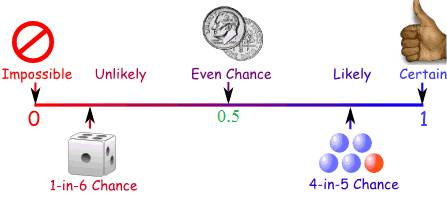The above picture summarises  chances of some thing happening from 'impossible' to 'possible'.

As we know ‘probability’ means chance of some thing happening. It could be any of the following:

1.    The chances of rains on any particular day

2.    The chances of India winning cricket test matches, one-day world cup

3.    A cricketer scoring century in a particular test match

4.    India winning Olympic medals in 400 meters relay

5.    Number of times  head comes up when  1 rupee coin is tossed  up 100 times

6.    Number of times  number 2 appears when a dice is drawn 500 times

The outcome of  any of the above event is pure chances. It may happen or it may not happen. Do you notice the difference between   first 4 statements and the last 2 statements? In case of first 4 cases, the result depends upon external factors such as place, time, strength of opposition team.. where as result of 5 and 6  will almost be same irrespective of place and time of the event.  There are cases when outcome of certain events can be forecast with some certainty. One such event is tossing of coin. When we toss the coin there can only be 2 outcomes, either head appears or tail appears. When coin is tossed up several times obviously, the chances of head coming up can vary from 0% to 100% and tail coming up can vary from 100% to 0%. However when  coin is tossed up in large number of times(in thousands)  head coming up may be 50% and tail coming up could be 50%.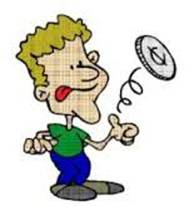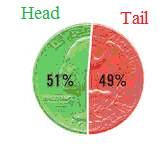In such cases, the probability is 1/2. Note that when head appears tail can not appear at the same time. Similarly when tail appears, head can not appear at the same time. This  we say that events are exclusive. That is to say, there can not be any other possibility other than head appearing or tail appearing. In such case, we note that the sum of their probability is 1/2+1/2= 1. Note that probability is expressed as a ratio and it is always ≤  1. why does dice have dots numbers from 1 to 6?.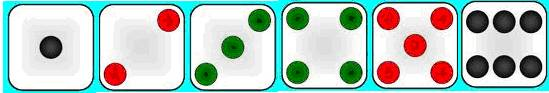Note that dice is a cube and it has 6 faces. Hence their faces are represented by 1 to 6 dots representing numbers from 1 to 6. When a dice is cast what are the chances of a number appearing from 1 to 6? It is one out of 6 and hence the probability is 1/6.

What is the sum of probabilities of number appearing between 1 and 6?

It is = Probability of number 1 appearing+ Probability of number 2 appearing + Probability of number 3 appearing + Probability of number 4 appearing + Probability of number 5 appearing + Probability of number  appearing between 1 and 6

= 1/6+1/6+1/6+1/6+1/6+1/6= 1

(Note that, when one number appears when a die is cast, other number can not appear at the same time. Since probability of each number appearing is excusive of others, sum of their probabilities is equal to 1.

Probability of number 1 not appearing

= Probability of number 2 appearing + Probability of number 3 appearing + Probability of number 4 appearing + Probability of number 5 appearing + Probability of number 6 appearing = 1/6+1/6+1/6+1/6+1/6= 5/6

This also can be calculated as

Probability of number 1 not appearing = 1 - Probability of number 1 appearing = 1-1/6= 5/6

Let us learn some of the definitions of terms used

 No Term Meaning Examples 1 Experiment Test or a procedure or operation which produces a result. In this lesson all experiments will be random. 2 Trial Performing an experiment tossing a coin/throwing a dice 3 outcome Result of trial head or tail/ appearance of number from 1 to 6. 4 Sample space Set of all possible outcomes S = {H,T}. S = {1,2,3,4,5,6} Event Every  subset of  Sample Space Getting the head=A= {H}, Getting the number 4=B={4} Getting even numbers= B = {2,4,6} 5 Elementary event Each of the outcome in sample space. That is  each of the element in sample space. (Every subset of sample space having only one element) Getting the head=A= {H}, Getting the number 4=B={4}

 Experiment Sample space and Events No of events n(S) Event A Favourable outcomes to event A and n(A) Probability P(A) = n(A)/n(S) 1 coin is tossed S={ H,T } 2 Tail coming up A=(T) n(A)=1 1/2 2 coins are tossed S={ HH,HT,TH,TT } 2*2=4 Tail should not come up A = {(HH)} n(A)=1 1/4 3 coins are tossed S={ HHH,HHT,HTH,THH, HTT,           THT,TTH,TTT } 2*2*2=8 Same face coming up A= {(HHH),(TTT)} n(A)= 2 2/8 1 dice is cast S={1,2,3,4,5,6 } 6 Even number coming up A={2,4,6} n(A)= 3 3/6 2 dices are cast S={(1,1),(1,2),(1,3),(1,4),(1,5),(1,6)         (2,1),(2,2),(2,3),(2,4),(2,5),(2,6)         (3,1),(3,2),(3,3),(3,4),(3,5),(3,6)         (4,1),(4,2),(4,3),(4,4),(4,5),(4,6)         (5,1),(5,2),(5,3),(5,4),(5,5),(5,6)         (6,1),(6,2),(6,3),(6,4),(6,5),(6,6)        } 6*6=36 Getting  the same number A= {(1,1),(2,2),(3,3), (4,4),(5,5),(6,6) n(A)= 6 6/36

P(E) = Probability of an event ‘E’ =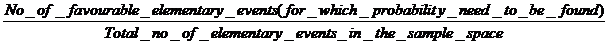=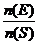Observe that P(A)+ P(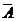) =1  (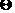n(A)/n(S) + n()/n(S) ={ n(A) + n()}/n(S) = n(S)/ n(S) )P(A) =1- P(), P() =1- P(A)

Probability: It is the ratio of the number of elementary elements favourable to the event E to the total number of elements in the sample space

Can probability ratio be 0 or 1?

1.    What is the probability of getting a number 0  or greater than 6 when dice is cast?

In this case  A = {} an empty set,  and n(S) = 6  , hence P(A)= n(A)/n(S)= 0 (When a  dice is cast number is  one among 1 to 6 only). This we call an impossible event, because such a event does not happen

2.    What is the probability of getting a number  1 to 6 when dice is cast?

In this case  A = S   and hence n(A) = n(S) = 6  , Thus P(A)= n(A)/n(S)= 1 (When a  dice is cast number is always  one among 1 to 6). This we call a sure  event, because such a event always    happens

Thus 0     P(A) ≤  1 This is what we represented in the beginning by a figure: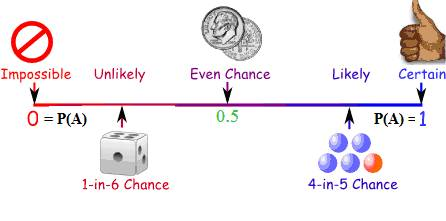Problem 1 : A survey of 850 working woman showed that 158 used own four-wheeler, 416 used two-wheeler, and the remaining used public transport. If one is chosen randomly among these women, what is the probability of the woman who commute by (1) own four wheeler (ii) Own two wheeler (iii) public transport and (iv) own vehicles.

Solution:

Number of women travelling by their own vehicles= 158+416= 574

Number of women travelling by public transport= 850-574= 276

Probability of woman using own four wheeler = 158/850

Probability of woman using own two wheeler = 416/850

Probability of woman using public transport = 276/850

Probability of woman using own vehicle      =   574/850= (158/850+416/850)

Problem 2 : A box  contains 12 balls out of which 'x' are red.

(i) If one ball is drawn, what is the probability of getting red ball?

(ii) If 6 more red balls are put in the box, the probability of a drawing a red ball will be doubled than earlier. Find 'x'

Solution:

(i)           Since 'x' number of balls are red out of  total of 12 balls, the probability of getting red is x/12

(ii)          When 6 more  balls are added, total number of balls become 18 (=12+6)

Hence the new probability of getting  a red ball = (x+6)/18

It is also given that new probability is twice the earlier probability thus New probability = 2x/12= x/6;(x+6)/18 = x/6

6x+36=18x

36= 12x

x=3

1.1 Summary of learning

 No Points studied 1 Probability,Event,Favourable event, exclusive Event